# CIFAR-100

CIFAR-100 computer-vision training dataset

The CIFAR-100 dataset contains 50,000 training and 10,000 test images of 20 object classes, along with 100 object subclasses. It is traditional to train on the 100 object subclasses. Each image is an RGB image of size 32x32.

(50000 elements)

## Examples

### Basic Examples

Retrieve the resource:

 In:=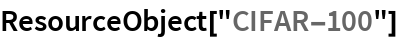Out=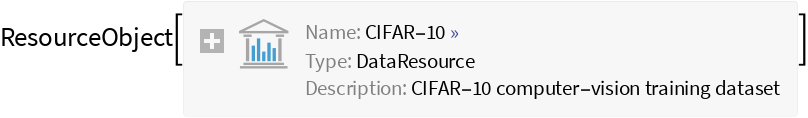View the data:

 In:=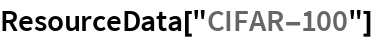Out=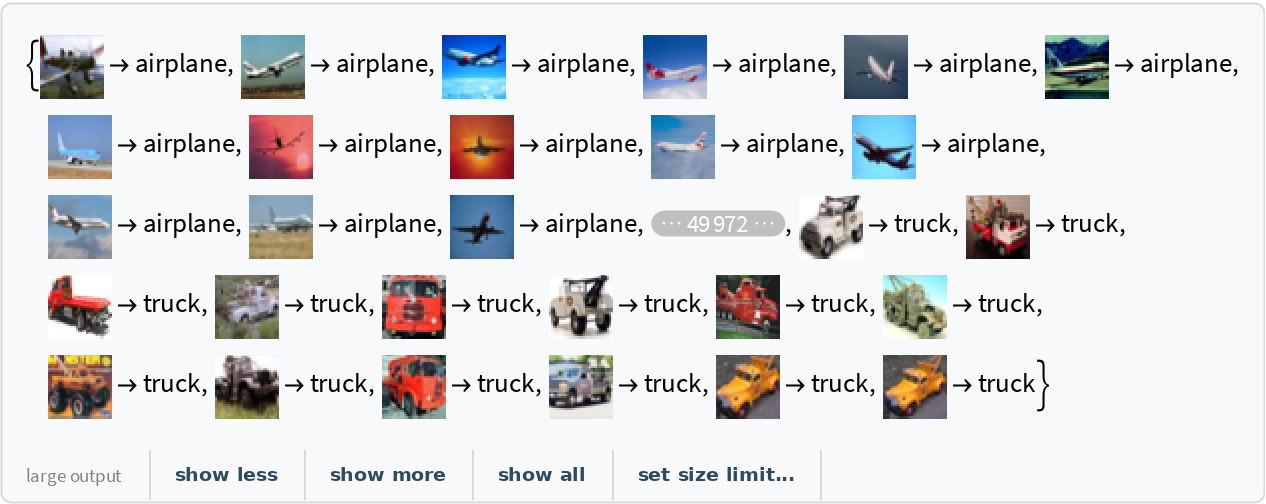### Analysis

View the list of classes in the dataset:

 In:=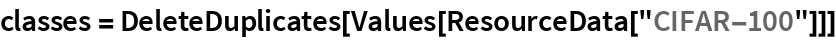Out=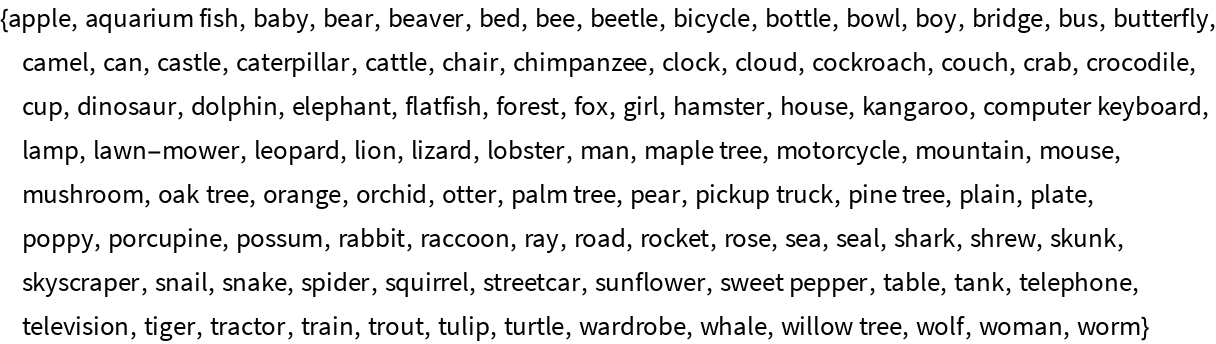Obtain one example of each class:

 In:=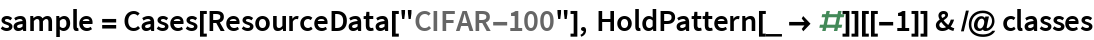Out=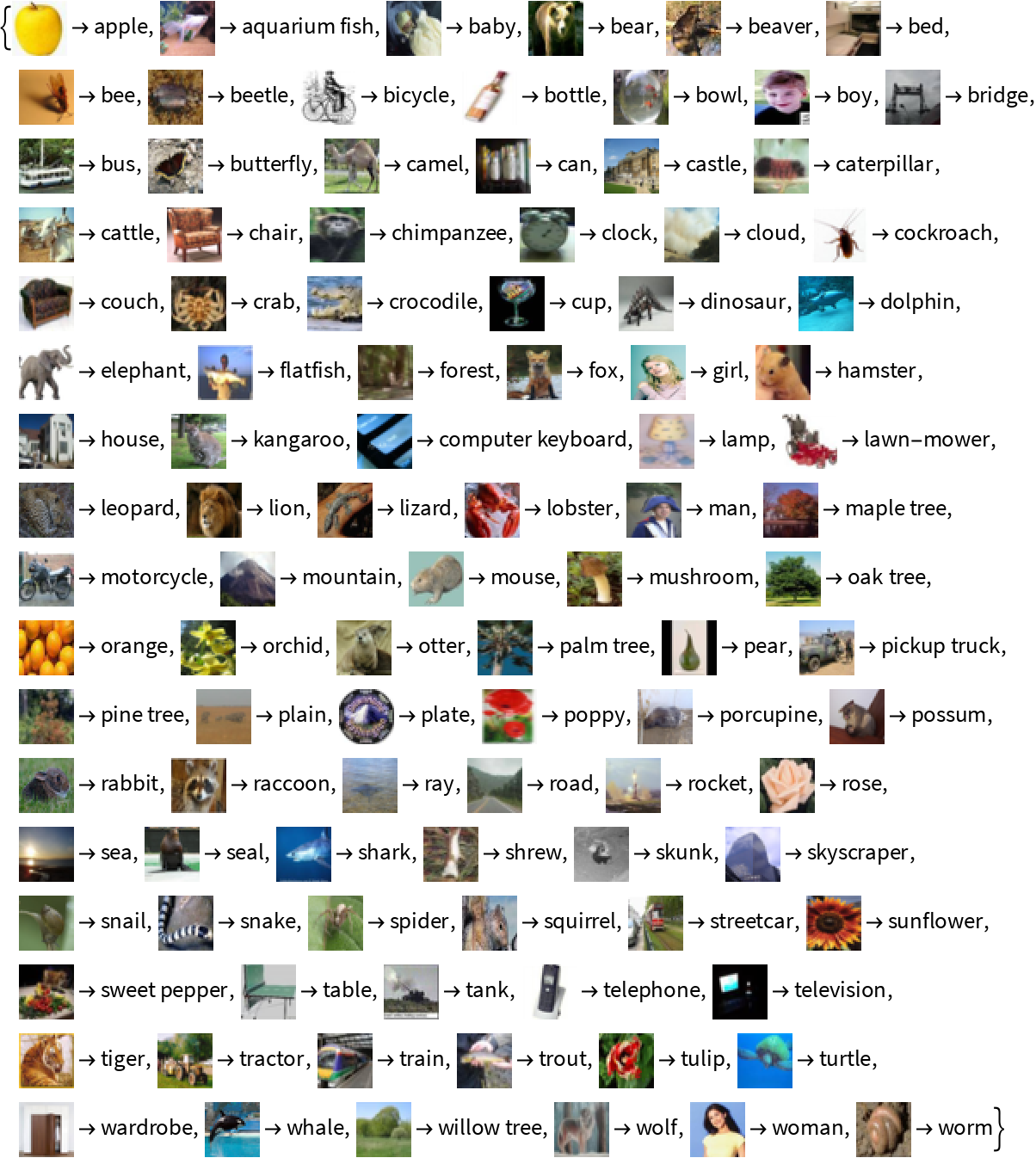Test the ImageIdentify function on an example image:

 In:=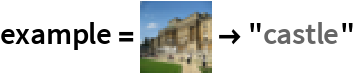Out=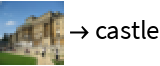In:=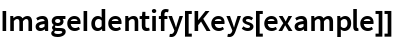Out=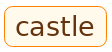Wolfram Research, "CIFAR-100" from the Wolfram Data Repository (2018)